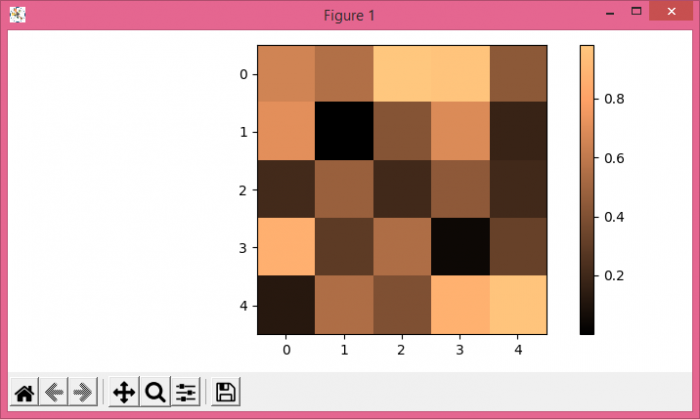# How do I change the font size of ticks of matplotlib.pyplot.colorbar.ColorbarBase?

To change the font size of ticks of a colorbar, we can take the following steps−

• Create a random data set of 5☓5 dimension.
• Display the data as an image, i.e., on a 2D regular raster.
• Create a colorbar with a scalar mappable object image.
• Initialize a variable for fontsize to change the tick size of the colorbar.
• Use axis tick_params() method to set the tick size of the colorbar.
• To display the figure, use show() method.

## Example

import numpy as np
from matplotlib import pyplot as plt
plt.rcParams["figure.figsize"] = [7.00, 3.50]
plt.rcParams["figure.autolayout"] = True
data = np.random.rand(5, 5)
im = plt.imshow(data, interpolation="nearest", cmap="copper")
cbar = plt.colorbar(im)
tick_font_size = 10
cbar.ax.tick_params(labelsize=tick_font_size)
plt.show()

## Output